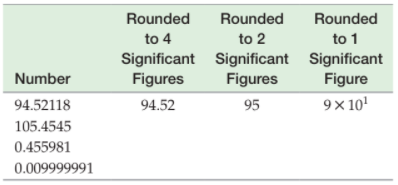×
Get Full Access to Introductory Chemistry - 5 Edition - Chapter 2 - Problem 56p
Get Full Access to Introductory Chemistry - 5 Edition - Chapter 2 - Problem 56p

×

# Round the number on the left to the number of significant figures indicated by theISBN: 9780321910295 34

## Solution for problem 56P Chapter 2

Introductory Chemistry | 5th Edition

• Textbook Solutions
• 2901 Step-by-step solutions solved by professors and subject experts
• Get 24/7 help from StudySoup virtual teaching assistantsIntroductory Chemistry | 5th Edition

4 5 1 295 Reviews
24
1
Problem 56P

Round the number on the left to the number of significant figures indicated by the example in the first row. (Use scientific notation as needed to avoid ambiguity.)Step-by-Step Solution:

Solution 56P

Step 1:

First let’s see the rules for significant numbers :

1. All non-zero digits are considered significant. For example, 81 has two significant figures- 8 and 1, while 123.45 has five significant figures -1, 2, 3, 4 and 5.
2. Zeros appearing anywhere between two non-zero digits are significant.

Example: 101.1203 has seven significant figures: 1, 0, 1, 1, 2, 0 and 3.

1. Leading zeros are not significant. For example, 0.00052 has two significant figures: 5 and 2.
2. Trailing zeros in a number containing a decimal point are significant. For example : 12.2300 has six significant figures: 1, 2, 2, 3, 0 and 0. The number 0.000122300 still has only six significant figures (the zeros before the 1 are not significant). In addition, 120.00 has five significant figures since it has three trailing zeros.

Step 2 of 2

##### ISBN: 9780321910295

Unlock Textbook Solution